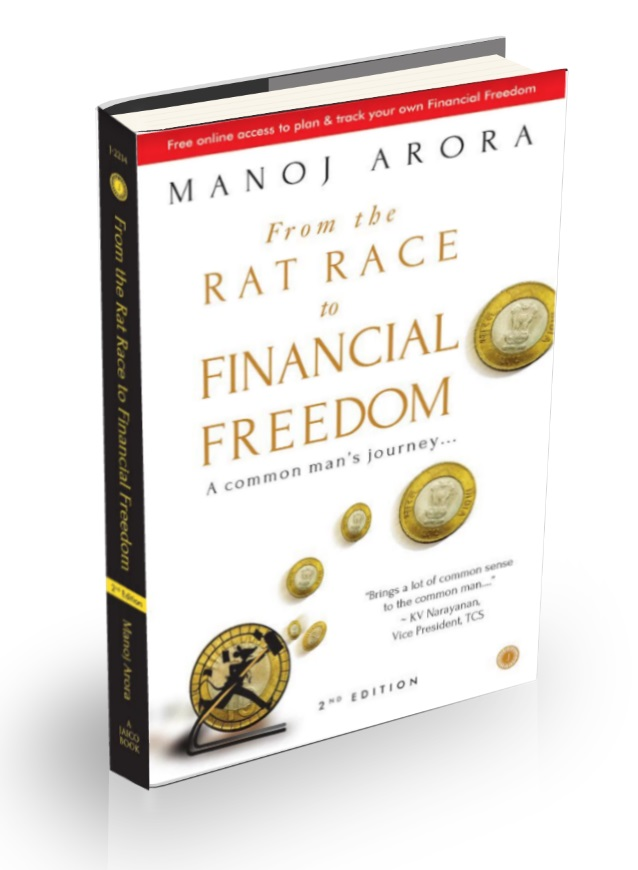Read Reviews >Buy a copy >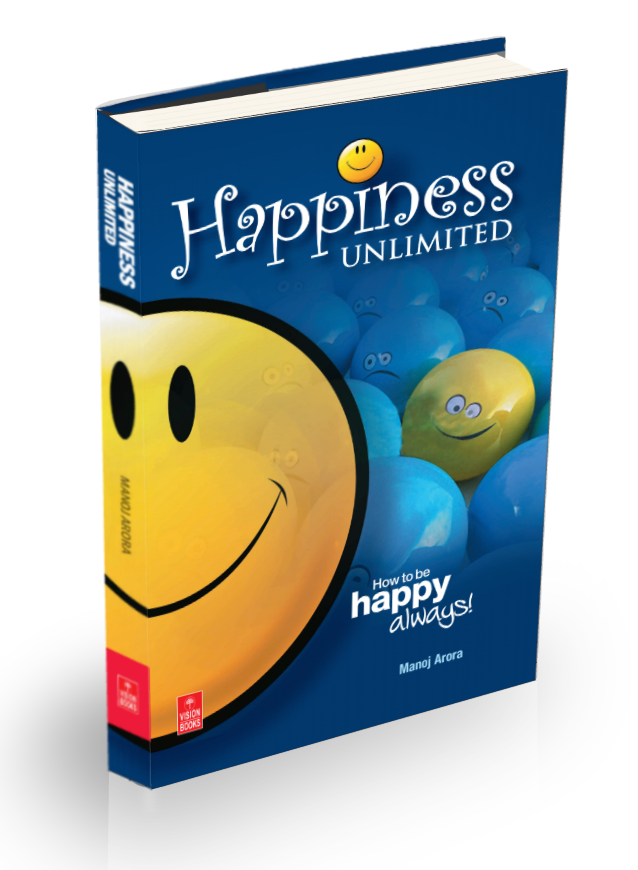Read Reviews >Buy a copy >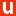Read Reviews >Buy a copy >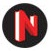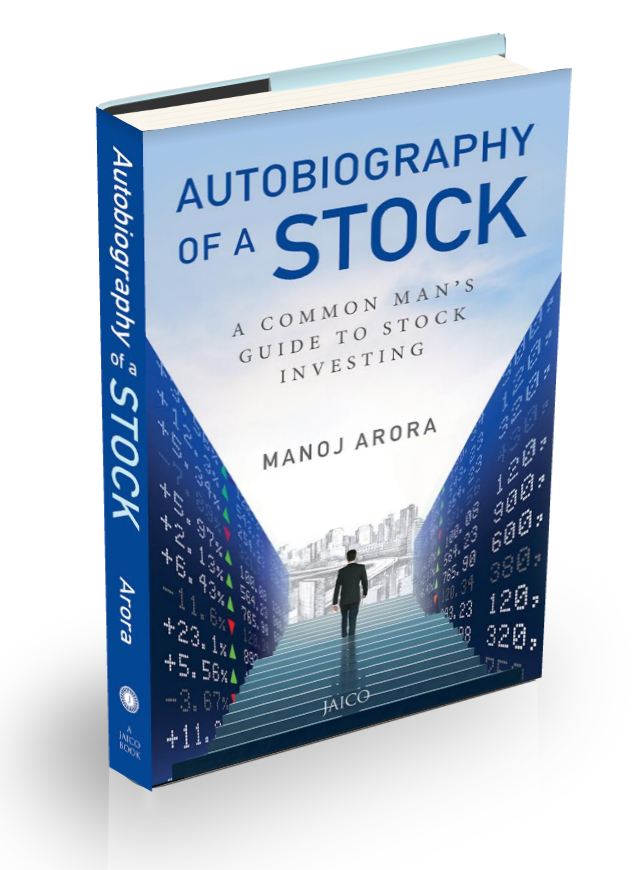Read Reviews >Buy a copy >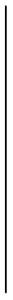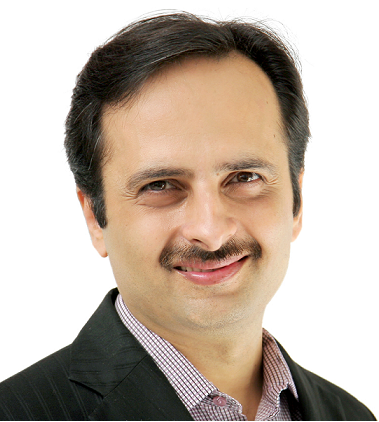About MeMy MissionAwards & CredentialsCorporate & Family Gifts [NEW]Visit Author's Page at AmazonVisit Author's Page at Flipkart

## Thursday, May 23, 2019

### KEEP INVESTING is an INEFFICIENT Approach to Wealth Building

A Mutual Fund SIP is supposed to be the simplest and most powerful investment option in the market even today. Though old fashioned, it needs almost negligible time investment from an investor post its setting up. But if you are just continuing to invest regularly, then you are missing one of the most vital aspects of wealth management...

Mutual Fund SIPs are a great long term tool for wealth generation. It has also been proven that once invested in SIPs, even the worst performing mutual funds in the market have generated positive returns over the long term.
[Recommended Post --> How long is long term in Equity?]

There are many alternatives to SIP based mutual fund investing today e.g. VIPs, which offer relatively better returns but needs your active intervention..
[Recommended Post --> VIPs perform better than SIPs]

And of course you have Stocks for those who have time to analyse companies, and more importantly the attitude to handle significantly higher volatility. There is no way Mutual Funds can match up to Stocks - not only in terms of returns but also in terms of enhancing your entrepreneurship skills.
[Recommended Post --> Stocks Vs Mutual Funds]

Irrespective of whatever investment you choose, there is one basic mistake that most investors do.
They KEEP INVESTING !!

What is 'Keep Investing' Approach?
This is what most financial advisers would guide you to do - to Keep Investing. That is what the entire concept of SIP is based on. Irrespective of market being up or down, keep investing a fixed amount of money. During upswing, you will buy lesser units and during downswing, you will buy more units from the same amount of money. In the end, you will generate average of market returns by taking average of market risks.

But over time, and with experience, I have realised that KEEP INVESTING is a less than optimal approach to wealth generation. Then what is the right approach?
The right approach is KEEP BALANCING !!

What is 'Keep Balancing' Approach?
Your portfolio consists of both 'Equity' and 'Non Equity' components. Equity Components primarily include Equity based Mutual Funds and Stocks. Non Equity portion could include anything other than these two i.e. FDs, PPF, EPF, Real Estate, Bonds etc. Keep Balancing is the art of regularly balancing your portfolio as the market fluctuates because of the inherent nature of the Equity portion of your portfolio.

How it works?
We will take a very simplified version of an example to illustrate the concept to you. We will run through the example, first with KEEP INVESTING Approach and then with KEEP BALANCING approach, and then study the difference between the two.

Example
Let us assume a portfolio which is being built with Rs. 1000 per month investment in Equity Portion and a similar Rs. 1000 per month investment in Non-Equity Portion.

Equity Portion investment
Equity Investment : Rs 1000 per month (or Rs. 12,000 per annum)
Starting Market Index : 100
Market Growth is assumed as follows:
Market Index after 1 year : 115
Market Index after 2 years : 145
Market Index after 3 years : 105
Market Index after 4 years : 140

Non Equity Portion Investment
investment of Rs 1000 per month (or Rs.12,000 per annum) growing @ 7% pa for all 4 years.

Let us evaluate how our portfolio grows in both the approaches.

'Keep Investing' Approach
After 1st year
Annual Increase in index = (115-100)/100 = 0.15
Equity Amount = 12,000*(1+0.15) =13,800
Non-Equity Amount = 12,000*1.07= 12,840
Total Portfolio = 26,640
Equity % = 13800/26640 = 51.8%

After 2nd year
Annual Increase in index = (145-115)/115 = 0.26
Equity Amount = (13,800+12,000=25,800)*1.26)=32,508
Non Equity Amount = (12,840+12,000)*1.07 = 26,578
Total Portfolio = 59,086
Equity % = 32,508/59,086 = 55.0%

After 3rd year
Annual Increase in index = (105-145)/145 = -0.28
Equity Amount = (32,508+12,000=44,508)*0.72)=32,046
Non Equity Amount = (26,578+12,000)*1.07 = 41,278
Total Portfolio = 73,324
Equity % = 32,046/73,324 = 43.7%

After 4th year
Annual Increase in index = (140-105)/105 = 0.33
Equity Amount = (32,046+12,000=44,046)*1.33)=58,581
Non Equity Amount = (41,278+12,000)*1.07 = 57,007
Total Portfolio = 1,15,588
Equity % = 32046/115588 = 50.7%

So, we end up with a portfolio of Rs. 1,15,588. Please note that though the equity % of the portfolio kept changing in between all these years but we ignored the fluctuation. We just kept investing a fixed amount of Rs. 12,000 every year and let the market take its own course to generate wealth.

'Keep Balancing' Approach
The first thing that we do in this approach is to decide on our Equity % balancing that we need in our portfolio.

Now, this is dependent on many factors including your age, life situations, risk capacity, risk capability, liquidity needs in future and so many other factors. We calculate this for all our freedom seekers enrolled for our ELITE program.
Let us say that we take 50% equity as the target for our example.

This means that whenever the markets go low, the portfolio balance will automatically fall below 50% equity (because your equity is growing at a much slower pace than debt), then you must buy more equity by withdrawing money from your debt so that the balance comes back to 50% (Buy Low)

This also means that whenever the markets go high, the portfolio balance will automatically move beyond 50% equity (because your equity is growing at a much faster pace than debt), you must sell from equity and load up your debt so that the balance comes back to 50% (Sell High)

This act of continuously balancing your portfolio is what is going to help you get the most optimum returns since it automatically follows the most fundamental equity investing principle - Buy Low & Sell High

Let us continue with the same example and how we should be approaching our investments with KEEP BALANCING approach

After 1st year
Annual Increase in index = (115-100)/100 = 0.15
Equity Amount = 12,000*(1+0.15) =13,800
Non Equity Amount = 12,000*1.07= 12,840
Total Portfolio = 26,640
Equity % = 13,800/26,640 = 51.8%

Re-balance the equity % to a fixed 50% by moving 1.8% funds to Non Equity portion
Surplus Equity = 1.8% of 26,640 = 480
Balanced Equity Amount = 13,800-480 = 13320
Balanced Non Equity Amount = 12,840 + 480 = 13320

After 2nd year
Annual Increase in index = (145-115)/115 = 0.26
Equity Amount = (13,320+12,000=25,320)*1.26)=28,840
Non Equity Amount = (13,320+12,000)*1.07 = 26,160
Total Portfolio = 55,000
Equity % = 28,840/55,000 = 52.4%

Re-balance the equity % to a fixed 50% by moving 2.4% funds to Non Equity portion
Surplus Equity = 2.4% of 55,000 = 1,340
Balanced Equity Amount = 28,840-1,340 = 27,500
Balanced Non Equity Amount = 26,160 + 1,340 = 27,500

After 3rd year
Annual Increase in index = (105-145)/145 = -0.28
Equity Amount = (27,500+12,000=39,500)*0.72)=36,140
Non Equity Amount = (27,500+12,000)*1.07 = 40,340
Total Portfolio = 76,480
Equity % = 36,140/76,480 = 47.2%

Re-balance the equity % to a fixed 50% by moving 2.8% funds to Equity portion
Surplus Equity = 2.8% of 76,480 = 2,100
Balanced Equity Amount = 36,140+2,100 = 38,240
Balanced Non Equity Amount = 40,340 - 2,100 = 38,240

After 4th year
Annual Increase in index = (140-105)/105 = 0.33
Equity Amount = (38,240+12,000=50,240)*1.33)=66,820
Non Equity Amount = (38,240+12,000)*1.07 = 53,760
Total Portfolio = 1,20,580
Equity % = 66,820/1,20,580 = 55.4%

Difference in Portfolio based on both the approaches
Now, compare the Total Portfolio after the end of 4th year in KEEP INVESTING Approach (INR 1,15,588) Vs the Total Portfolio in KEEP BALANCING Approach (INR 1,20,580). This is an awesome difference of 4.3% in returns.

A 4.3% higher returns can do wonders to your portfolio. This can mean many things.
- This could mean pulling your financial freedom year forward by many years - thus allowing many more years to work on your passion.
- This could mean earning 14% returns instead of 10%.
- This could mean earning an additional passive income of Rs. 35,000 per month on a corpus of 1 Crores.

It could mean so many different things to different investors.

Summary
While KEEP INVESTING Approach is the most rudimentary approach necessary for wealth generation, you must move to KEEP BALANCING Approach as you get smarter and wiser. Make sure, you hit the right Equity % balance and get it calculated by an expert. This % is very customised for every freedom seeker. Once done, enjoy the fruits of passive income flowing into your corpus while you work to make your life more meaningful.

Regards

Manoj Arora
Official Website

1.Another insightful article from Manoj. The compounding effect of portfolio balancing approach compared to regular investing is really stupendous. I have got the flavour of the effect myself as an ELITE program member.

1.Thanks and Cheers...

2.The post is pretty much valid for the balancing part, but i wonder why is the example just shown comparing equity vs non equity (FD/PPF/EPF/RealEstate) and why not Debt funds which can also give as high as 10% cagr? Views are welcome from the writer.

1.Dear Sneha

Wonderful question.

The example is a simplistic view to enable seekers to get the concept right.
As long as their is volatility in market - which swings the market more than the returns from non equity portion, portfolio balancing will always fetch better returns than just continuing to keep investing - even if the non equity portion contains instruments with 10% returns.

Regards

Manoj